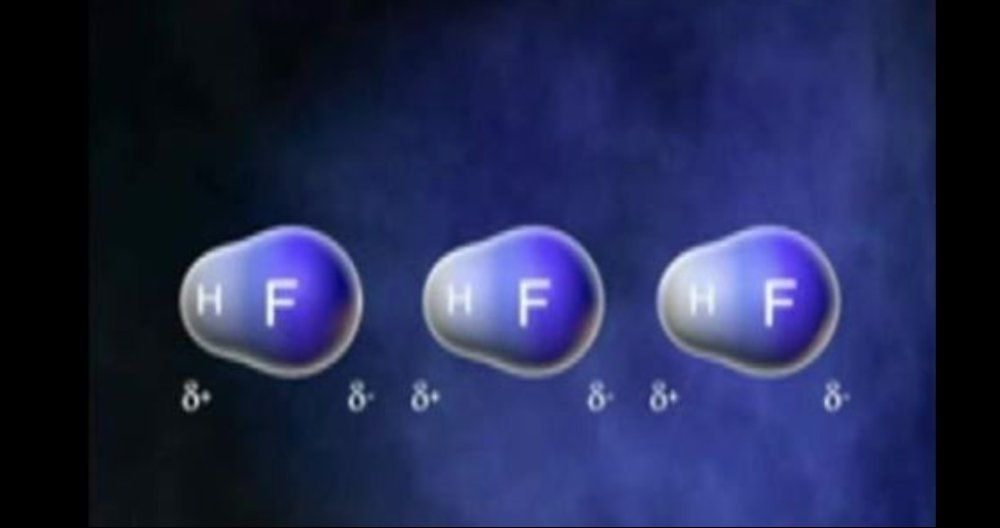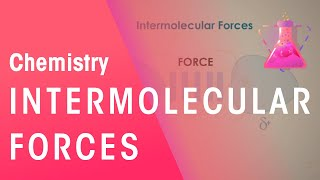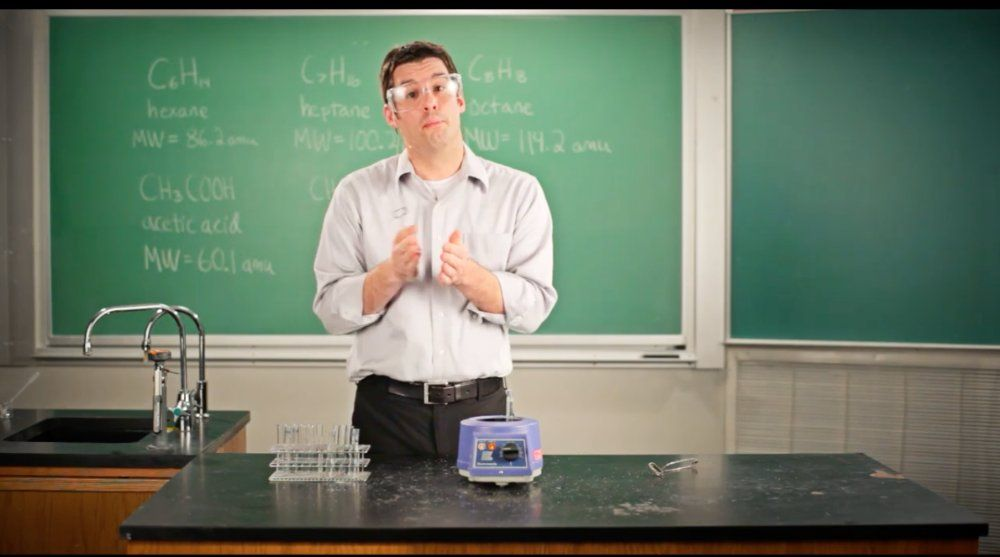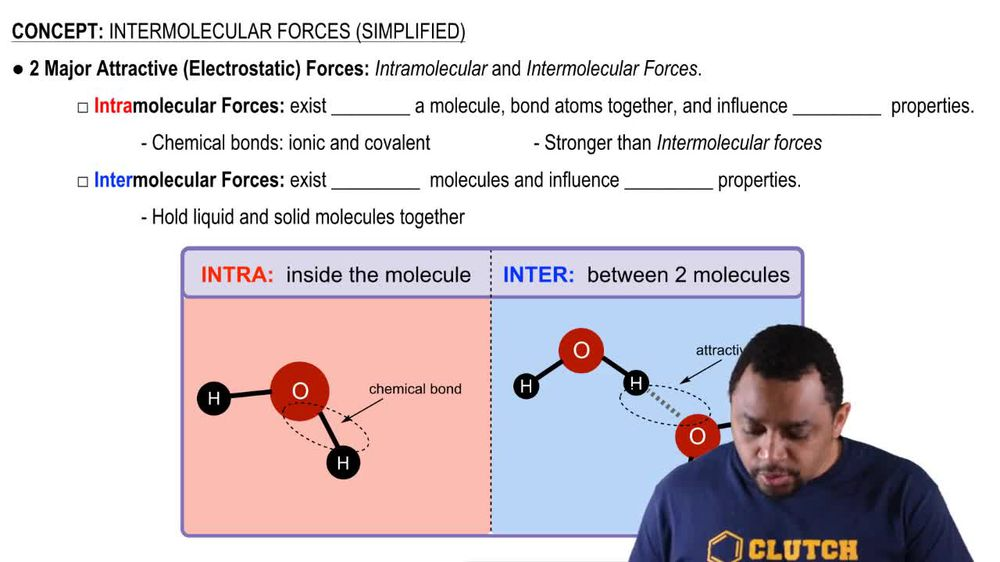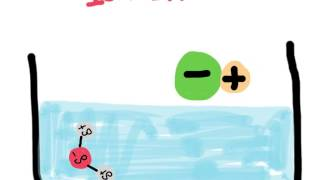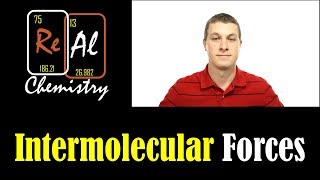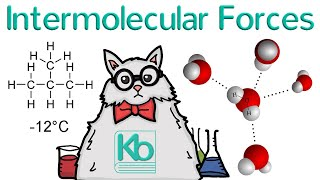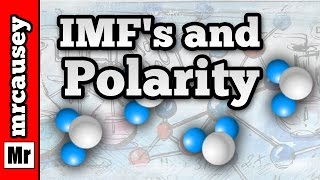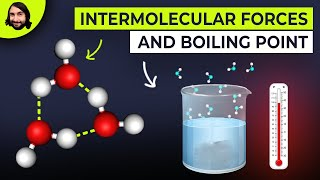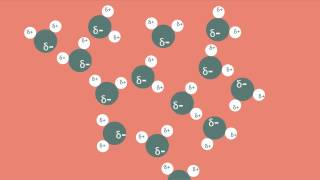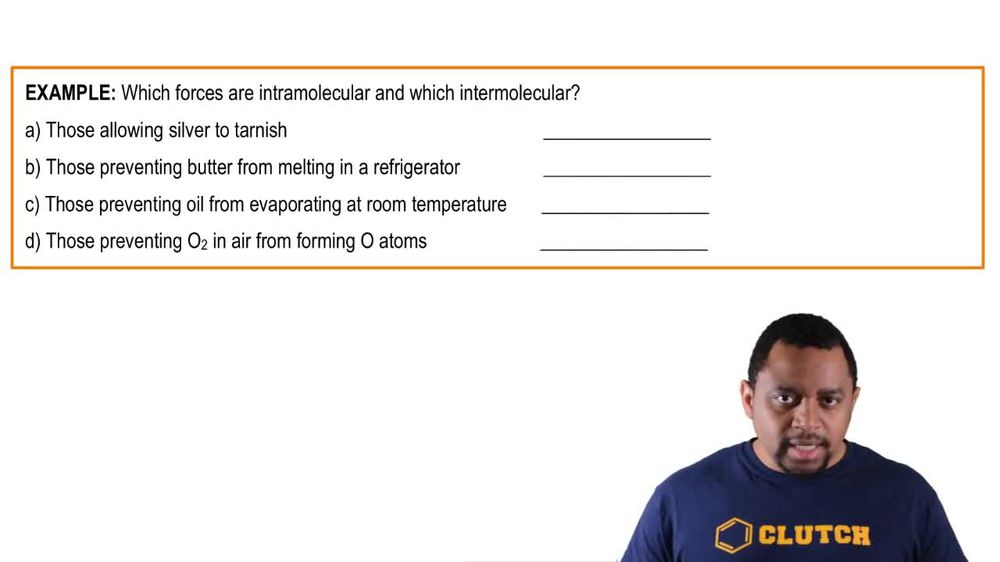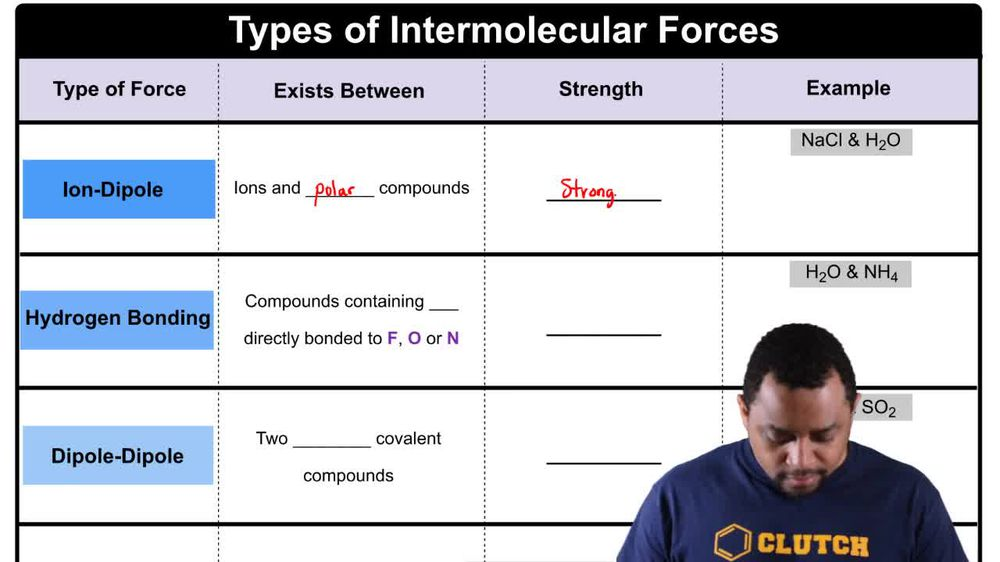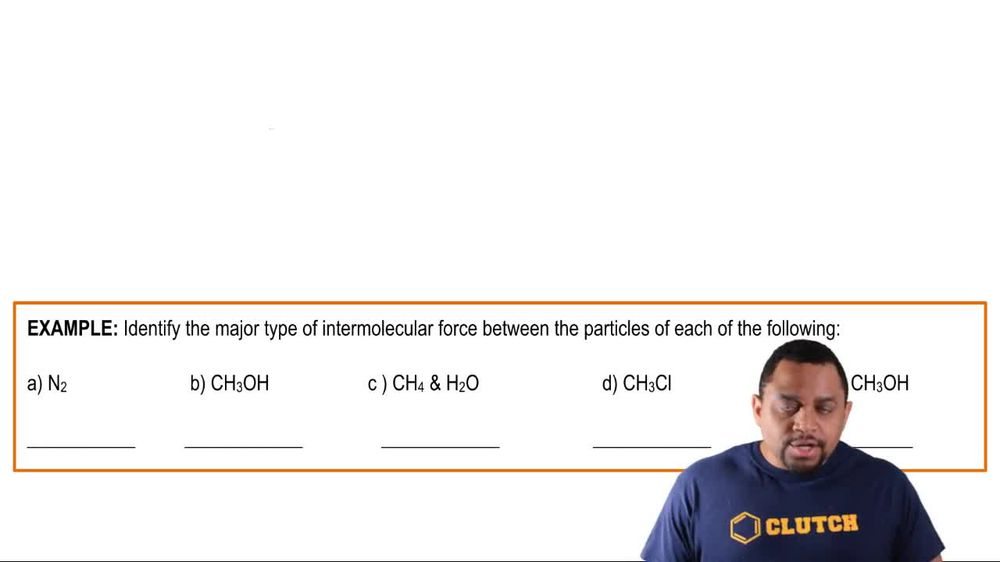Start typing, then use the up and down arrows to select an option from the list.
1. 13. Liquids, Solids & Intermolecular Forces2. Intermolecular Forces# Intermolecular Forces Concept 2

by Jules Bruno
253 views
5
0
when talking about the types of inter molecular forces, realize that there are four types of them and they are the forces that attract molecules together. Now here we're going to say polarity of compounds plays a big role in the type of force present. So let's go through this. We have our types of forces. Then we have the exist between. We talk about their strengths and then we give an example. So the first time we have is ion dipole. This is the force that exists between ion so positive and negative ions and polar compounds. They are the strongest of the inter molecular forces. So anytime you see an ionic compound, you can assume that water is the solvent that it can be dissolved in. Okay, usually you'll see ionic compound with water but let's say you see only an ionic compound and they ask what's the inter molecular force. Just assume it's dissolved in water. When we dissolve sodium chloride and water, we have ions and a plus Equus and cl minus acquis remember a quiz, just means that ion is submerged in water. And what's actually happening is that we have our sodium ion here, which is positive. The oxygen end of water which is partially negative, would be attracted to it because opposite charges attract the attractive force would be illustrated by this dotted line. See all minus is negative. So it would be the hydrogen end of water that would be attracted to it because water would be partially positive here. And the attractive force between chlorine and hydrogen will be symbolized by that dotted line. So this is what we mean. When we say an ion is a quiz in solution, it's water molecules surrounding it, basically dissolving it. We're gonna say solving it. These are terms that we usually use. So i on diaper is the strongest force. It's indicative of an ionic compound being dissolved within a poor substance, such as water. The next one is hydrogen bonding. This is when we have compounds containing hydrogen directly connected to fund fon flooring, oxygen and nitrogen. So here this one is the second strongest after ion dipole. Here we have water and we have NH four. NH four is a poly atomic ion. It's NH four positive, it's the ammonium ion. We could show water molecules Being attracted to one another. Yeah. And the dotted lines show that attraction. Or we could show NH four positive molecules being attracted to one another. Or actually we can show them being attracted to the water. So hydrogen years partially positive oxygen and its lone pair would be attracted to one of these hydrogen. So it's those dotted lines between these molecules that represents the hydrogen bonding. But hydrogen bonding itself can't exist unless the molecule is showing an attractive force between hydrogen flooring oxygen or nitrogen. The next one is dipole dipole. Now, before we talk about diapering diaper realized that hydrogen bonding is a special type of dipole dipole. It's also dipole dipole itself is just hydrogen bonding type of dipole dipole. This is when you have two polar koval, it compounds? This one here is the third strongest of all the inter molecular forces. So here we have Hcl which is polar and S. O. To which is polar. And here we could show the attraction between them. So remember chlorine has its lone pairs, it's more electro negative than hydrogen. So it'll be partially negative, hydrogen will be partially positive Within S. 02. You could show the sulfur with its lone pairs, oxygen with its lone pairs, oxygen is more electro negative. So it be partially negative. It would form an attractive connection to the hydrogen of the hcl. That dotted line would be the dipole dipole interaction between these two molecules. And it could only happen because both molecules are polar. Finally, we have the last and the weakest inter molecular force which is called London dispersion. And sometimes it's called dispersion forces and sometimes it's called Van der Waals Forces. This is the dominant force between two non polar covalin compounds. Here we have CH four which is a hydrocarbon and here we have CCL four which is non polar as well. Now if you don't know this, make sure you go back and take a look at my videos on molecular polarity where we show how to draw these structures and explain why exactly they're non polar. So here you have CH four And here we have CCL four. Now both of these are non polar. So why exactly would they all of a sudden be attracted to one another? Well that's because when molecules come too close together there is an instance because of their close proximity to one another that they developed a slight polarity. That slight polarity between each other causes them to become attracted to one another. So we'd say that there is some type of attraction between this molecule and this molecule because of that instance that moment where one being too close to another makes them both slightly polar for a moment. Overall they're still non polar though. We're just talking about that split moment. Now these are our rankings of the inter molecular forces. And what's important though here is that the London dispersion forces, although they're the weakest, they are actually present in all types of compounds. So water which has its main inter molecular forces being, hydrogen bonding actually has some London dispersion forces in it. Mhm. This ionic compound being dissolved in water, it's major forces ion dipole, but it also has a little bit of London dispersion forces in it as well. So just realize that although dispersion forces are the weakest, they are president all compounds, so they serve an important purpose